# Code Daily – Tutorial – Fundamentals of Rendering Data as an SVG Line with D3 and scaleLinear

By Prosyscom
In March 17, 2018
109 Views

## Intro

Visualizations can be intimidating. We’ll be focusing on the fundamentals. Nothing crazy, just drawing a line. We’ll explain everything that goes into taking your data, rendering it and making sure it fits inside your SVG, and then we’ll throw a little curve at the end.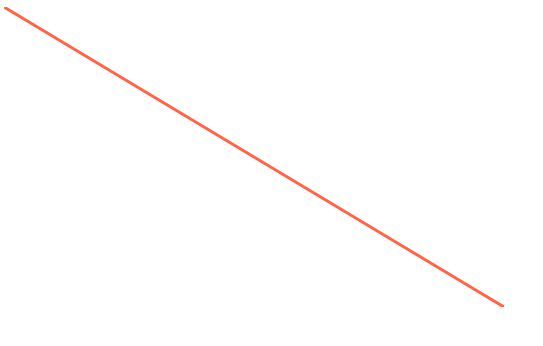## Install Dependencies

First off D3 is the defacto standard for visualizations. It makes it easy to handle all the math, path building, etc and let React handle the rendering of it. We will use 2 packages from d3, the `d3-shape` library for building up our `line` path. Then the `d3-scale` that will handle the translation of our data to renderable numbers.

``npm install d3-shape d3-scale``

## Data

Our data is structured specifically for this tutorial. The `x` is just increasing by 1 each time and the `y` is increasing by 50 each time. This will cause nice steps and will be easy to show off how the path structure is actually built and translated from our data.

``````export const data = [
{
x: 0,
y: 0,
},
{
x: 1,
y: 50,
},
{
x: 2,
y: 100,
},
{
x: 3,
y: 150,
},

{
x: 10,
y: 500,
},
];``````

## Selectors

These are our data selectors, just JavaScript functions. These aren’t special to D3 but it’s what we’ll use to select the piece of data for each `x` and `y` pointer.

``````const xSelector = d => d.x;
const ySelector = d => d.y;``````

Imagine if `y` wasn’t your key but instead it was `price`. Well rather than `d => d.y` you’d have used `d => d.price`. That way you don’t have to pre-process your data and add `x` or `y` properties, we just use some selectors to grab values for us.

## Setup SVG

First off we’ll define a width/height. When dealing with SVGs knowing the width/height is crucial. We’re defining it as 500 wide and 300 high. This will give us the ability to scale our data down.

``````const width = 500;
const height = 300;``````

The outer wrapping `<div>` isn’t necessary. The `svg` is given our `width` and `height`. We’ll then place our svg paths inside of it.

``````return (
<div>
<svg width={width} height={height}>

</svg>
</div>
);``````

## scaleLinear

First off we need to import `scaleLinear` from `d3-scale`.

``import { scaleLinear } from "d3-scale";``

`scaleLiner` provides a way to create a `range` and a `domain` that are proportionally mapped. When give a value it has the ability to extrapolate in either direction. Mostly you are providing a `value` that is within the `domain` you have set. `scaleLinear` then extrapolates (fancy word for “does some math”) and figures out what the output value is based on the `range` you’ve specified. Generally speaking the `range` will be the dimensions of the SVG you are rendering into.

The reason we need to scale our data is because the values of your data don’t necessarily coordinate to actual rendering pixels. Our SVG is 500×300, but what if it were 800×500? Or 100×30? The data is still the same but we need to extrapolate where each data point should be rendered based on the size of our SVG.

To read more about `scaleLinear` you can see what the documentation says about it here d3-scale#scaleLinear.

So for our `xScale`. We are rendering from `0` to the `width` of our SVG which is 500. Our domain of our data is the minimum x value and the maximum x value. The positions in the array for the range, coordinate to the positions of the domain.

So if you call `xScale` in this instance and give it the value `0`. It will return your `0`. If you call it with the value `10`, it’s going to return you the `500` aka the width. Now if you passed in `5` it will see that it is halfway between `0` and `10` in the domain, and extrapolating between `0` and `500` will return `250`.

The `x` axis is the horizontal axis, and will help us position our rendering left <=> right.

``````const xScale = scaleLinear()
.range([0, width])
.domain([0, 10]);``````

So you can see here that all this `scaleLinear` is doing is some math based upon your dimensions (range) and your data (domain). We’ve manually specified the array `[0, 10]` for our domain but this can be programmatically figured out using a `min` and or `max` function.

Now we’re onto our `y` axis which is our vertical axis which will help us in our rendering top <=> bottom.

``````const yScale = scaleLinear()
.range([0, height])
.domain([0, 500]);``````

Our data range for our `y` parameter extends from `0` to `500`. So just like before we setup our `domain` based upon our data.
The `range` is setup based upon our height, which is `0` to `300` in this case.

For the `x` our data was easy it was numbers between 1 and 10. This is numbers between 0 and 500.

``````value => output
0 => 0
50 => 30
100 => 60
150 => 90
...``````

Our data is being scaled down this time to fit within the constraints of our `300` height.

Overall if you are rendering some graph data you’ll likely use `scaleLinear` to map your domain values to the range values to render within your svg.

## Build a Line

Now we need to build up our line. So we’ll use the `line` function from `d3-shape`.

``import { line } from "d3-shape";``

We need to create a `line` and give an `x` and a `y`. What is going to happen is that our `path` that we create will eventually be given some data. Once it does it will loop over it and for each data point it called our `x` and `y` functions we specified.

Our `x` and our `y` first call their appropriate selectors. This will pluck off the value from each of our object structures that is structured like so `{ x: 1, y: 50}`. Then we’ll call our `scaleLinear` functions we setup. These will take the data value, and scale it to our renderable value. Then we’ll return that to our line so it can figure out how we want our line created.

``````const path = line()
.x(d => xScale(xSelector(d)))
.y(d => yScale(ySelector(d)));``````

Finally we call it with our data, and it will render our line. Don’t forget we need to give it a `stroke` of color, a `strokeWidth` which we set to 3, and tell it `fill="none"` otherwise it’ll fill in with black.

``````return (
<div>
<svg width={width} height={height}>
<path d={path(data)} stroke="#ff6347" strokeWidth={3} fill="none" />
</svg>
</div>
);``````

## Path

If we take a look at what `path(data)` spits out it might be more clear about what’s happening.

``M0,0L50,30L100,60L150,90L200,120L250,150L300,180L350,210L400,240L450,270L500,300``

Those values sure do look familiar! Hey those are the exact values we were figuring out in the `scaleLinear` section up above.

The first is an `M` which will move to our first position from our data`0,0`.
Then our next will draw a line to using `L` to our `x` and `y`. This is `x=1` and `y=50` in our data world. When mapped through our domain to our range it output `50,30`. Rendering a line to the point `x=50` and `y=30`.## Flip It

When dealing with graphing `0` is the bottom left corner. However when dealing with SVG `0` is the top left corner. That’s why when we our rendering our values it’s starting in the top left corner.

We told it via our `domain` and `range` that `0` should map to `0`.

``````.range([0, height])
.domain([0, 500]);``````

Well if we wanted our `0` value to actually start at the bottom left corner we need to flip around range around.
So 0 value is actually mapped to our `height` aka the bottom left corner, and our maximum value is actually `0` the top right.

``````const yScale = scaleLinear()
.range([height, 0])
.domain([0, 500]);``````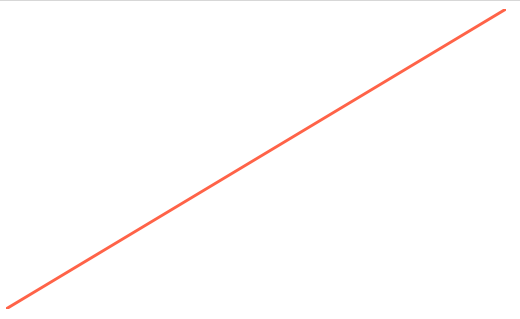## Up and Down Data

We can also render different data. Lets map a pyramid like route, it’ll go up then down.

If you import the data

``import { data as downUpData } from "./down_up";``

It’ll look like this.

``````{
x: 3,
y: 150,
},
{
x: 4,
y: 200,
},
{
x: 5,
y: 250,
},
{
x: 6,
y: 200,
},
{
x: 7,
y: 150,
},``````

Rather than the straight line data we need to fix our `path` call to take in our `downUpData`.

``<path d={path(downUpData)} stroke="#ff6347" strokeWidth={3} fill="none" />``

You can see that the `path` we created was just instructions on how to render data. It wasn’t tied to any specific data.
We can swap the data out and get a completely different result!

Now depending on what point you choose for your range either `[0, height]` or `[height, 0]` will change where it renders at.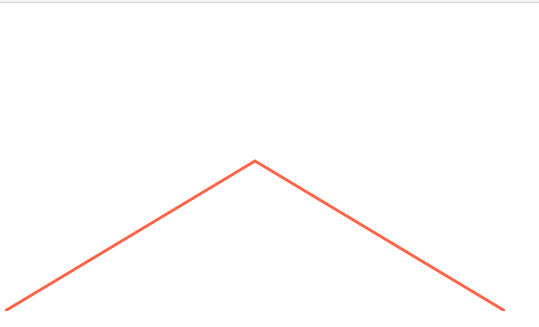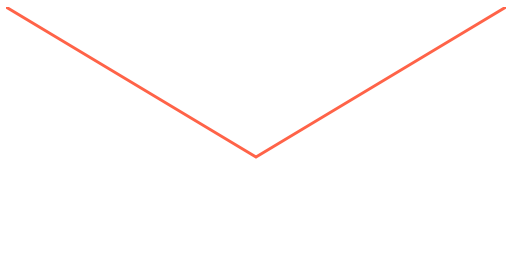## Different Curves

One final note is you can specify how the data `curves`. This will instruct the `line` how to build out the actual path to render.

We need to import it from `d3-shape` along with our `line`.

``import { line, curveStep } from "d3-shape";``

Then rather than just an `x` and a `y` we supply a `curve`. This will give a function that will effect how the final output path is constructed.

``````    const path = line()
.x(d => xScale(xSelector(d)))
.y(d => yScale(ySelector(d)))
.curve(curveStep)``````

You can see here there are a few more instructions compared to the previous path.

``M0,0L25,0L25,30L75,30L75,60L125,60L125,90L175,90L175,120L225,120L225,150L275,150L275,120L325,120L325,90L375,90L375,60L425,60L425,30L475,30L475,0L500,0``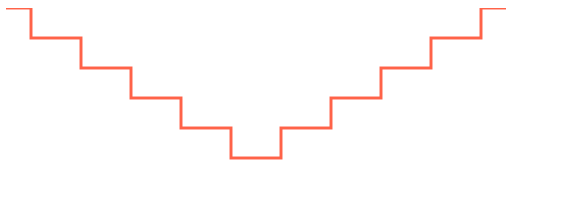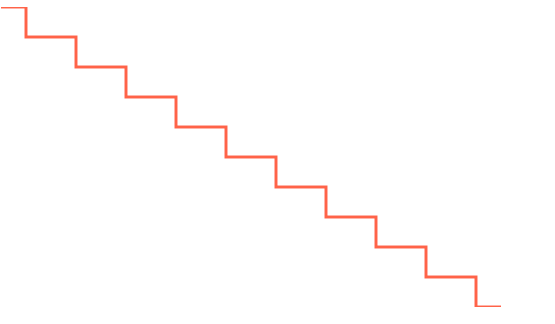## Ending

Change around the `width/height`, see how the path is effected, see what it does to your line and how it renders.